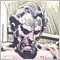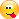# Determine if three doubles have the same sign3512

Here's what I have so far, and it works, at least it seems to.

Is there a better way to do this?

Also not 100% sure of the 0 condition.

I'm interested in any commentary that generates an interesting, more efficient, and/or more elegant function in MQL5.

```// Check if three doubles have the same sign.
bool HaveSameSign(const double a, const double b, const double c)
{
// 0 condition - A zero doesn't have a sign.
if ( a == 0. || b == 0. || c == 0. )
{
return false;
}

// Checks signs
if ( a > 0. && ( b < 0. || c < 0. ) ) return false;
if ( a < 0. && ( b > 0. || c > 0. ) ) return false;

return true;
}```19663

`return (a>=0 && b>=0 && c>=0) || (a<0 && b<0 && c<0);`38895

Anthony Garot:

Here's what I have so far, and it works, at least it seems to.

Is there a better way to do this?

Also not 100% sure of the 0 condition.

I'm interested in any commentary that generates an interesting, more efficient, and/or more elegant function in MQL5.

`        // 0 condition - A zero doesn't have a sign.`

That's not right, IEEE754 (double) has signed zero. But I don't think you have to bother about it unless you want to discriminate +0 and -0.

Which I do for fun```//+------------------------------------------------------------------+
//| Union to work with some "double" bits                            |
//+------------------------------------------------------------------+
union _64bits
{
private:
double            d;
long              l;
public:
void   _64bits(double v) : d(v)  { };
void operator=(double v)         { d=v; };
long sign(void) const            { return(l>>63); };
};
//+------------------------------------------------------------------+
//| True of all values in the array have same sign, otherwise false  |
//+------------------------------------------------------------------+
bool HaveSameSign(double &arr[])
{
int size=ArraySize(arr); if(size==1) return(true);
//--- first element
_64bits test(arr);
long sign=test.sign();

//--- if a sign is different
for(int i=1;i<size;i++)
{
test=arr[i];
if(sign!=test.sign())
return(false);
}
//--- all values with same sign
return(true);
}
const int COUNT = 3;
const int SHIFT = 32768/2;
//+------------------------------------------------------------------+
//| Script program start function                                    |
//+------------------------------------------------------------------+
void OnStart()
{
MathSrand(GetTickCount());
double testcase[];
ArrayResize(testcase,COUNT);

for(int z=0;z<10;z++)
{
for(int i=0;i<COUNT;i++)
{
testcase[i]=(MathRand()-SHIFT);
}
ArrayPrint(testcase);
printf("SAME SIGN : %s",HaveSameSign(testcase) ? "YES" : "NO");
}
}```
-0.,-0.,-0.   SAME SIGN : YES
+0.,+0.,+0.  SAME SIGN : YES
+0.,-0.,+0.  SAME SIGN : NO3512

Alain Verleyen:

That's not right, IEEE754 (double) has signed zero. But I don't think you have to bother about it unless you want to discriminate +0 and -0.

Which I do for fun

-0.,-0.,-0.   SAME SIGN : YES
+0.,+0.,+0.  SAME SIGN : YES
+0.,-0.,+0.  SAME SIGN : NO

Impressive! You compare the sign bit directly, and you allow for any number of values to be checked.

Thanks for the IEEE754 article. Interesting, but as you say, probably nothing I need to both about. :-D3512

Forum on trading, automated trading systems and testing trading strategies

Determine if three doubles have the same sign

William Roeder, 2019.08.15 01:59

`return (a>=0 && b>=0 && c>=0) || (a<0 && b<0 && c<0);`

Simple, clean, and direct.3512

Out of curiosity, I wanted to see how MQL handles +0. and -0. values.

It appears:

1. They can be assigned simply as +0. and -0.

2. Testing with the == operator says they are the same.

And the only way to know this is using Alain's union.

```#property strict

//+------------------------------------------------------------------+
//| Union to work with some "double" bits                            |
//| (Added operator== to Alain's code)                               |
//+------------------------------------------------------------------+
union _64bits
{
private:
double            d;
long              l;
public:
void _64bits(double v) : d(v)    { };
void operator=(double v)         { d=v; };
bool operator==(_64bits &v)      { return d==v.d && l==v.l; };
long sign(void) const            { return(l>>63); };
};

void OnStart()
{
// See how MQL handles +/- 0.
double a = 0.;
double b = -0.;
if ( a == b ) Print("same"); else Print("not same");

_64bits c(a);
_64bits d(b);
if ( c == d ) Print("same"); else Print("not same");

PrintFormat("sign a [%d] sign b [%d]", c.sign(), d.sign() );
}```
2019.08.15 08:38:11.876    negative zero (GBPJPY,H4)    same
2019.08.15 08:38:11.876    negative zero (GBPJPY,H4)    not same
2019.08.15 08:38:11.876    negative zero (GBPJPY,H4)    sign a  sign b [-1]875

This is even shorter:

`return !(((a>=0) + (b>=0) + (c>=0))%3);`

Although it's just to show that it can be done that short in MQL. The one by @William Roeder is easier to read and maintain.

Some other solutions:

`return MathAbs(a)+MathAbs(b)+MathAbs(c)==MathAbs(a+b+c);`
`return MathMin(MathMin(a,b),c)>=0 || MathMax(MathMax(a,b),c)<0;`

Excluding zeroes as originally requested:

`return a*b>0 && b*c>0;`3512

Some other solutions:

These are clever. Nicely done. I like the last one best.